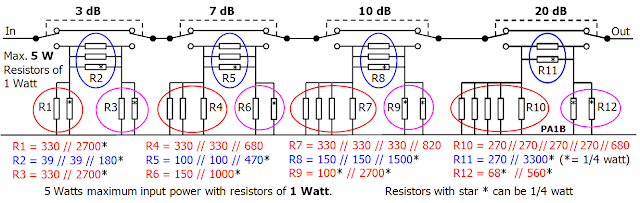### PA1B Fast and accurate 40 dB Power Attenuators for QSO's

A simple way to reduce the power of your QRP set to milliwatt level, without modifying the set, is the use of attenuators. These attenuators are designed to reduce the power of a QRP set with a power of 5 watts.

Maximum power of the resistors
To reduce the number of resistors, the attenuator can be built with resistors of 2 watts of 1 watts. The higher the maximum power of the resistors, the less resistors are needed. The attenuator with 2 watt resistors is designed for a nominal input power of 1.5 watts. (Max. 6 W)

PA1B Fast and accurate 40 dB Power Attenuator for QSO's
for a nominal input power of 1.5 watts with metal film resistors of 2 watts. (2018)PA1B Fast and accurate Power Attenuator for QSO's  Nominal power 1.5 W (Max. 6 W) (2018)   PA1B
Fast in QSO's
A simple way to reduce the power of your QRP set to milliwatt level, without modifying the set, is the use of attenuators. The combination of 3 dB, 7 dB, 10 dB and 20 dB is very fast to switch, when you want to increase your power in QSO's. In one move, one attenuator section is switched OFF and an other is switched ON, to increase the power with a step of 3 db or 4 dB.

Power of the attenuator
All attenuator sections are designed for an nominal input power of 1.5 watts. This is a maximu power of 6 W. The attenuator sections consist each of 3 compound resistors, which each are formed by a two or three resistors in parallel. These resistors are a power resistor of 2 watts and a some resistor of a modest power (1/4 watt or 1/2 watt). The resistors with a star * can be 1/4 watt. The resistors with a 2 stars ** can be 1/2 watt. Or you can chose 2 watt resistors for all resistors.
The power resistors, with a lower ohmic value, dissipate most of the power and an other resistor is added in parallel, to arrive accurately on the wanted ohmic value. The power attenuator sections of 10 dB and 20 dB are build with 8 resistors, since they must dissipate much more power than the other sections. Notice that all the power, that is not delivered to the output, must be dissipated by the attenuator.

Accuracy
With two or more resistors from the E12-series in parallel, any value can be made accurately.
In practice the accuracy of the resistors will be about 1.5%, so the accuracy of the attenuator is very good.
Don't be surprised, if the accuracy of the 20 dB and 10 dB sections is 0.05 dB and the other sections even better.

Simple layout
The choice of placing resistors in parallel, leads to a very simple layout of the attenuator sections.
There's NO need for a PCB.

PA1B Fast and accurate 40 dB Power Attenuator for QSO's
for a nominal input power of 1.3 watts with resistors of 1 watts. (2018)Fast and Accurate Power Attenuator for QSO with resistors of 1 watt. Nominal power 1.3 W. (Max 5 W)
The attenuator built with 1 watt resistors is designed for a nominal input power of 1.3 watts.

Power of the attenuator
All attenuator sections of this attenuator are designed for a nominal input power of 1.3 watts.
This is 5 watts in CW.
The attenuator sections consist each of 3 compound resistors, which each are formed by a number of resistors in parallel. These resistors are a power resistor of 1 watts and a some resistor of a modest power (1/4 watt). The resistors with a star * can be 1/4 watt. Or you can chose 1 watt resistors for all resistors.
The power resistors, with a lower ohmic value, dissipate most of the power and an other resistor is added in parallel, to arrive accurately on the wanted ohmic value. The power attenuator sections of 20 dB and 10 dB are build with 9 resistors, since they must dissipate much more power than the other sections.

Asymmetrical attenuator
Please notice that this attenuator is asymmetrical.
Input and output can not be exchangedTable for the fast and accurate  3 - 7- 10 - 20 dB    1.3 Watt  Power Attenuator